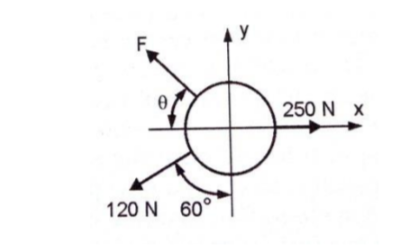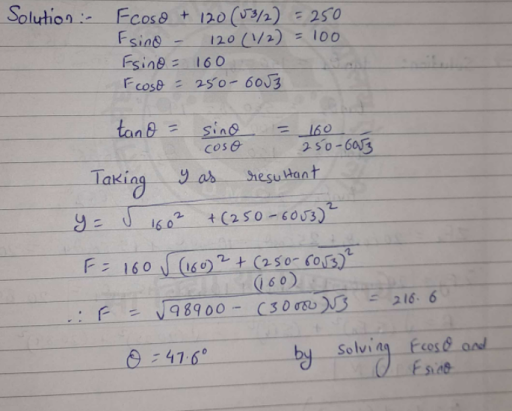# A ring is pulled by three forces as shown in figure. Find the force F and the angle θ if resultant of these three forces

more_vert

A ring is pulled by three forces as shown in figure. Find the force F and the angle θ if resultant of these three forces is 100 N acting in vertical direction.more_vert

verified

$$\theta=47.6^o$$